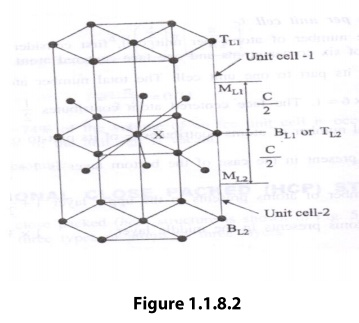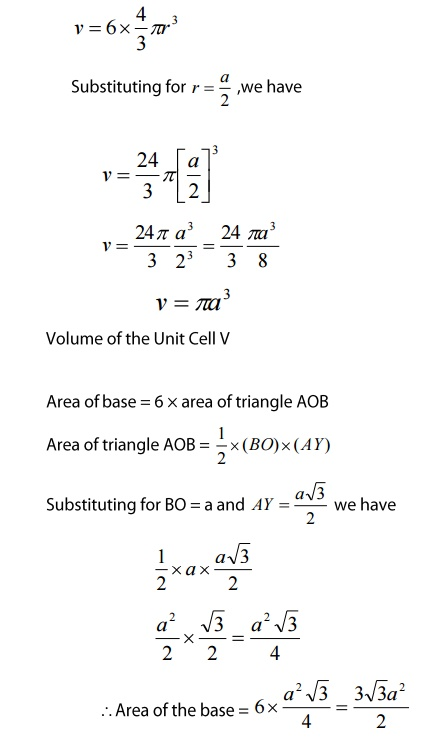Home | | Engineering Physics I | Hexagonal Closely Packed Structure(HCP)

# Hexagonal Closely Packed Structure(HCP)

The hexagonal closely packed (hcp) is shown in the figure 1.1.8. In the hcp structure of an unit cell contains three types of atoms as three layers.

Hexagonal Closely Packed Structure(HCP)

The hexagonal closely packed (hcp) is shown in the figure 1.1.8. In the hcp structure of an unit cell contains three types of atoms as three layers.12 corner atoms, one at each and every corner of the Hexagon.

2 base centered atoms, one at the top face of the hexagon and another at the bottom face of the hexagon.

In addition to corner and base atoms, 3 atoms are situated in between the top and bottom face of the hexagon, in alternate vertical faces. Also note that these atoms are situated inside the face so that they can’t be shared by other cells as shown in the figure .1.10

(i)                Number of atoms per unit cell

To calculate the number of atoms per unit cell, first consider the bottom layer. The bottom layer consists of six corner atoms and one face centered atom. Each and every corner atom contributes 1/6 of its part to one unit cell. The total number atoms contributed by the corner atoms is 1/6 x 6 = 1. The face-centered atom contributes ½ of its part to one unit cell. Therefore, the total number of atoms contributes ½ of its part to one unit cell. Therefore, total number of atoms present in the case of the bottom layer is 1 + ½ = 3/2

Similarly, the number of atoms present in the upper layer is 1 + ½ = 3/2

The number of the atoms present in the middle layer = 1 x 3 = 3

The total number of atoms present in the unit cell = 3/2 + 3/2 + 3  = 6 atoms.

To find the atomic radius of the hcp structure, consider any two-corner atoms. It has to be noted that, each and every corner atom touches with each other, therefore they are nearest neighbor.

From figure 1.1.8.1 we can write(iii)           Coordination Number

The coordination number of the hcp structure can be calculated as follows. Let us consider two unit cells as shown in the figure 1.1.8.2The hcp structure is considered to have three layers viz, 1) Bottom Layer [B1],2) Top Layer [T1] and 3) Middle Layer [M1] as shown.

In the top and bottom layers, the base centered atom is surrounded by six corner atoms. In the middle layer we have 3 atoms stacked inside the unit cell as shown. Let us consider two unit cells let ‘X’ be the reference atom taken in the bottom layer BL1 of unit cell 1(or top layer [TL2] of unit cell 2). This atom has 6 neighboring atom in its own plane. Further at a distance of c/2 it has 3 atoms in the middle layer (ML1) of unit cell -1 and 3 more atoms in the middle layer (ML2) of unit cell -2. Therefore, the total number of neighboring atoms is 6+3+3=12

Thus the coordination number is 12.We know that ‘c’ is the height of the unit cell of HCP structure and ‘a’ is the distance between two neighboring atoms. Now consider a triangle ABO in the bottom layer.

Here A,B, and O are the lattice points and exactly above these atoms at a perpendicular distance ‘c’/2 the next layer atom lies at C.(iv)           Atomic Packing factor

Volume of all atoms in a unit cell(v)

No of atoms per unit cell, n =6

Volume of all the six atoms in the unit cellThe volume of the unit cell of the HCP = base area x heightThus the packing density is 74% and hence, it is a closed packed structure.

Example. Zinc, Beryellium and Magnesium

Study Material, Lecturing Notes, Assignment, Reference, Wiki description explanation, brief detail
Physics : Crystal Physics : Hexagonal Closely Packed Structure(HCP) |